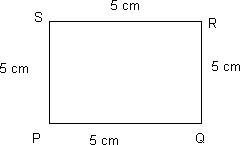Home >> Square >>

Define square - It is a quadrilateral

Area of square Perimeter of Square Diagonals of Square Construction of Square with Compass

Definition : A Square is a quadrilateral whose :-
1. All angles are of 90 Degree (right angled at each vertex)
2. Opposite sides are parallel to each other
3. All sides are of equal lengthAngles P, Q, R and S = 90 degree
Line PQ = QR = RS = SP = 5 cm
Line PQ // RS
Line PS // RQ
Hence, we can say that quadrilateral PQRS is a square.